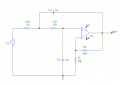# 2nd order filter output amplitudes

#### matt20

Joined Dec 3, 2020
2
Afternoon,

If I have a 2volt input for the below circuit (LM741 opamp), the gain would be 1+586/1000 = 1.586 giving me an output of 3.172 voltsSo 20 log (vout/vin) = log(1.586) = 0.2

Therefore 20*0.2 = 4db on the pass band as per the AC analysis.

So for the corner frequency I calculated as

3.172*0.707 = 2.24volts

Therefore 2.24/2 =1.121302 = log(1.121302) = 0.0492

Therefore 20*0.0492 = 1db as per the AC analysis.

But on the transient analysis the output is 2.14v not 2.24v at 1Khz, what causes this voltage drop ?

The same is for at 3Khz, the attenuation is -15.152db on the AC analysis

But when I calculate results from transient analysis I get,

20log (326mv/2) so (326mv/2) = 0.163, therefore log (0.163) = -0.7878

Therefore 20*-0.7878 = -15.75db.

thanks matt.

#### Papabravo

Joined Feb 24, 2006
20,607
Two things:
1. What is the actual magnitude of the errors?
2. What is the value of VC and VE?
It is well known that the 741 is a very poor performer at lower power supply voltages. The output cannot even begin to approach the supply rails. IMHO, for the signal levels you are using ±10VDC supplies would be the minimum.

#### Ian0

Joined Aug 7, 2020
8,947
159Ω would be difficult for a 741 to drive. Try it again with 10nF and 15.9k, and see if that makes a difference. (And check the supply rails).
And if it’s just a SPICE simulation, you can afford a better op-amp!

#### ericgibbs

Joined Jan 29, 2010
18,234
hi matt,
This is what I see in LTS.
E
Look at the box data lower right. 2.41V

AC Analysis

Last edited:

#### matt20

Joined Dec 3, 2020
2
Hi thank you for your replies. So Im using microcap software for my simulation. At fc which is around 1k, theres a 0.1v (2.14 to 2.24) difference from my calculated to the actual on the simulation results.
And at 3KHz the transient simulation shows the output amplitude at 326mV, but to give me the -15.2db as per the AC analysis it should be around 347mV (20log(0.34731/2)=~15.2db.

What is the reason for these differences? Is it due to losses within the opamp its self.
Hope that all makes sense, sorry I'm still learning.

#### Audioguru again

Joined Oct 21, 2019
6,444